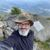QlikView App Dev

Discussion Board for collaboration related to QlikView App Development.

Announcements
QlikWorld 2022, LIVE in Denver CO., May 16-19, 2022. REGISTER NOW TO RECEIVE EARLY BIRD PRICING
cancel
Showing results for
Did you mean:Contributor II

Expression - double if

I've tried but haven't got the correct result.

I have a quantity unit "BOX" where I need to divide my quantity with 1000 and it works if I use the following expression:

sum   (   if (Unit = 'BOX' , Quantity/1000 , Quantity)  )

In addition I have two types of transactions, one is the cancellation so I would need to have this negativ. I use the following formula which works.

sum  (  if  (  Type = '648' , Quantity*-1 , Quantity)    )

How can I best combine them?

Thanks

1 Solution

Accepted SolutionsPartner

Hi,

not sure I understood, but something like:

sum   (   if (Unit = 'BOX' , Quantity/1000 ,

if  (  Type = '648' , Quantity*-1 , Quantity)

)  )

is what you wish?

S.

5 RepliesPartner

Hi,

not sure I understood, but something like:

sum   (   if (Unit = 'BOX' , Quantity/1000 ,

if  (  Type = '648' , Quantity*-1 , Quantity)

)  )

is what you wish?

S.Master III

sum   (   if (Unit = 'BOX' , Quantity/1000 ,if  (  Type = '648' , Quantity*-1 , Quantity)    ))Contributor II
Author

Thanks for that. The else statement confused me and I've tried something far to complicated. Appreciate the quick response.Specialist

Sum(if(...)) is as far from best practice as you can come.

This is much better to do in the script in my opinion.

if(Unit = 'BOX', Quantity/1000,if( Type = '648',Quantity*-1,Quantity) as NewQuantity

from Fact....;

Then the expression becomes Sum(NewQuantity)Contributor II
Author

Have solved it like this now. Thanks.Community Browser